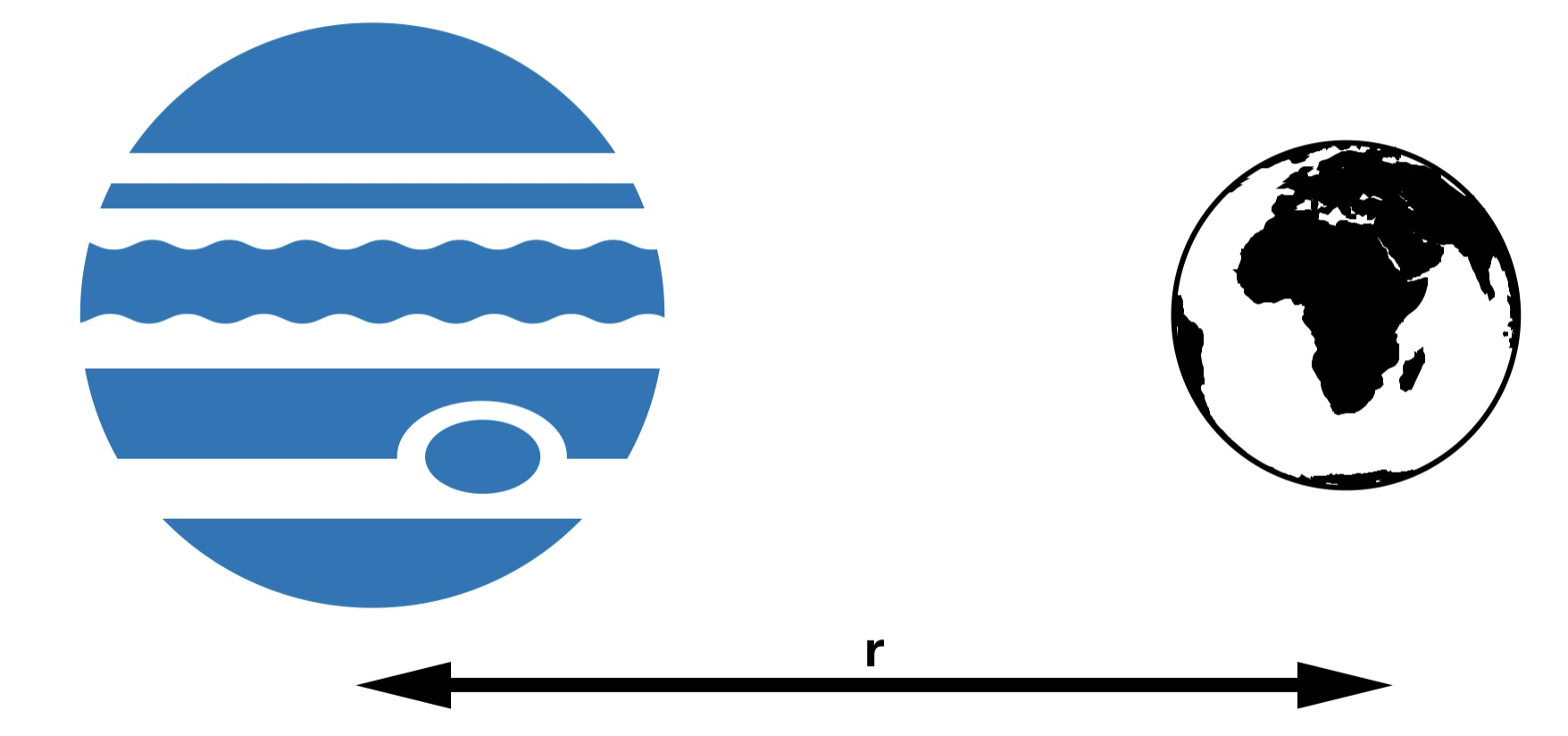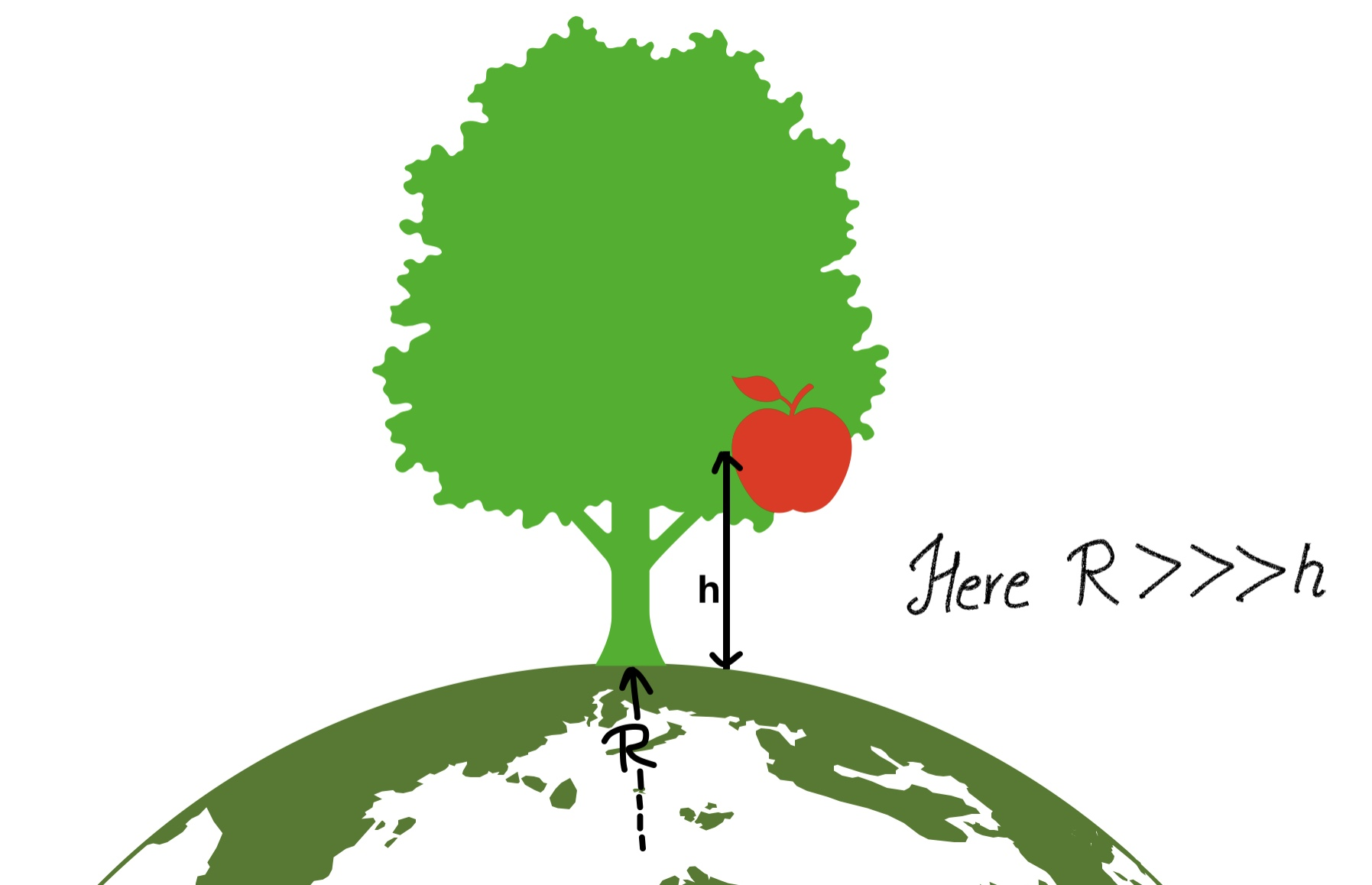# undamental Forces in nature

Fundamental Forces :- All the forces observed in nature such as muscular force, tension, reaction, friction, elastic, weight, electric, magnetic, nuclear, etc., can be explained in terms of only following four basic forces :-

(1) Gravitational Force

(2) Electromagnetic Force

(3) Nuclear Force

(4) Weak Force

##### (1) Gravitational Force

The force of interaction which exists between two particles of masses m1 and m2, due to their masses is called gravitational force.$\displaystyle F=G\frac{{{m}_{1}}{{m}_{2}}}{{{r}^{2}}}$

G : Universal Gravitational Constant = 6.67 × 10-11 Nm2/Kg2

m1,m2 : masses of the bodies

r : distance between the centers of the bodiesProperties of Gravitational force:-

(i) It is the weakest force.

(ii) It is always attractive in nature.
(iii) It is a long range force as it acts between any two particles situated at any distance in the universe.
(iv) It is independent of the nature of medium between the particles.

Now let us consider that an apple is freely falling as shown in figure:-when it is at a height h, force between earth and apple is given by :-$\displaystyle F=G\frac{{{M}_{e}}m}{{{({{R}_{e}}+h)}^{2}}}$

this force acts along the center of earth

Me : Mass of Earth

But here Re>>>>h, hence (Re + h) ≅ Re

So,$\displaystyle F=G\frac{{{M}_{e}}m}{{{({{R}_{e}}+h)}^{2}}}$  ≅$\displaystyle G\frac{{{M}_{e}}m}{{{({{R}_{e}})}^{2}}}$  =$\displaystyle m\left[ \frac{G{{M}_{e}}}{R_{e}^{2}} \right]$   …………………(1)

Comparing this equation with

F = ma  …………………(2)

we get$\displaystyle a=G\frac{{{M}_{e}}}{R_{e}^{2}}$

this quantity GMe /(Re)2 is called acceleration due to gravity and it is given a symbol “g”, hence acceleration due to gravity:-$\displaystyle g=G\frac{{{M}_{e}}}{R_{e}^{2}}$

using the values

G  = 6.67 × 10-11 Nm2/Kg2

Me = 5.972 × 1024 kg

Re 6.4 × 106 m

we get

g = GMe /(Re)2 = 9.8 m/s2 =  π2m/s2 = 32 ft/s2

g ≅ 10 m/s2

It is called acceleration due to gravity near the surface of earth.

##### (2) Electromagnetic Force

Force exerted by one particle on the other because of the electric charge on the particles is called electromagnetic force.

Following are the main characteristics of electromagnetic force:-

(i) These can be attractive or repulsive.
(ii) These are long range forces.
(iii) The value of these forces depend on the nature of medium between the charged particles.
(iv) All macroscopic forces (except gravitational) which we experience as push or pull or by contact are electromagnetic, i.e., tension in a rope, the force of friction, normal reaction, muscular force, and force experienced by a deformed spring are electromagnetic forces. On microscopic level these are electromagnetic attractions and repulsions between atoms/molecules.

##### (3) Nuclear Force

It is the strongest force in nature. It keeps nucleons (neutrons and protons) together inside the nucleus inspite of large electric repulsion between protons. Radioactivity, fission, and fusion, etc. result because of unbalancing of nuclear forces. It acts within the nucleus that too upto a very small distance.

##### (4) Weak Force

It acts between any two elementary particles(proton or neutron). Under its action a neutron can change into a proton emitting an electron and a particle called antineutrino. The range of weak force is very small, in fact much smaller than the size of a proton or a neutron.

##### comparision of magnitude of fundamental forces

Let us consider that two protons are placed at a distance of 1 Fermi (10-15m) from each others, then the ratio of  Nuclear force, Electromagnetic force, Weak force and Gravitational force between them is found to be…

FN : FEM : FW : F::  1 : 10-2 :10-7 :10-38